# Improving initial generations in pso algorithm for transportation network design problem

ijcsit
9 de Mar de 2015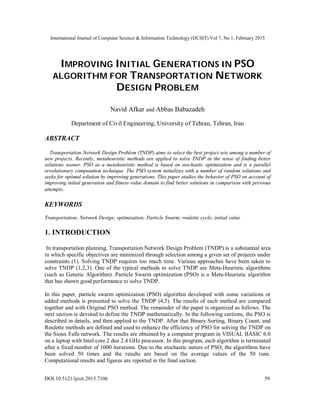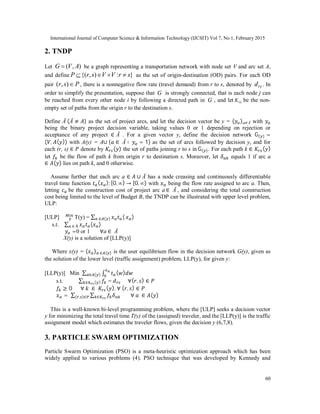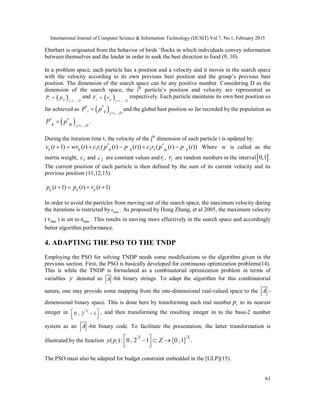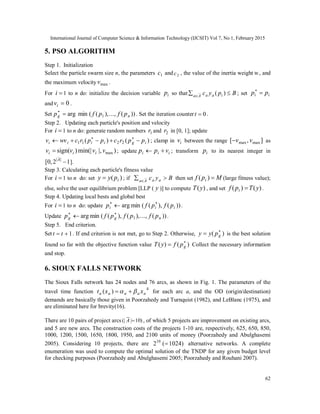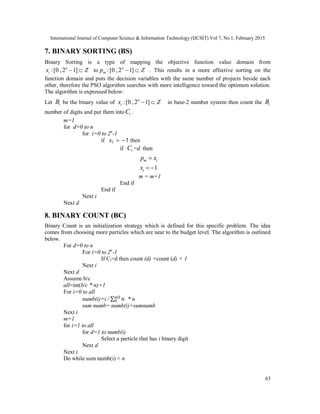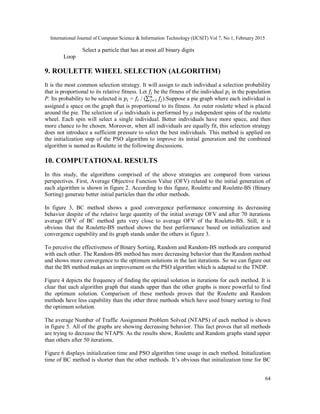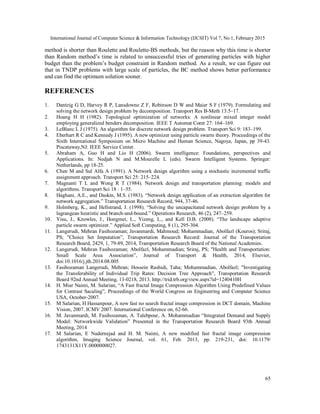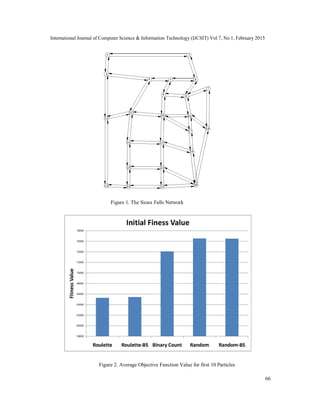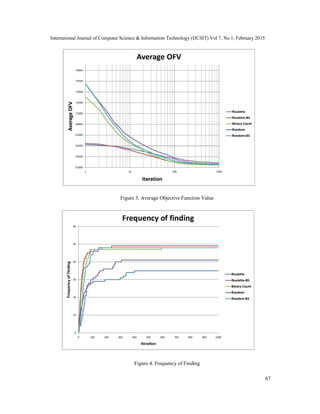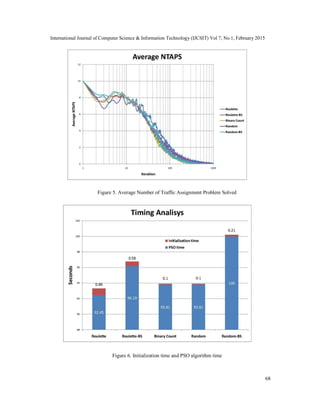Próximo SlideShare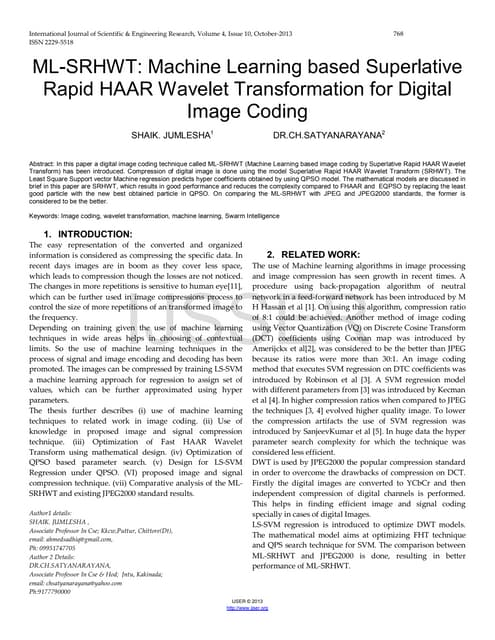Ml srhwt-machine-learning-based-superlative-rapid-haar-wavelet-transformation...
Cargando en ... 3
1 de 10

### Improving initial generations in pso algorithm for transportation network design problem

1. International Journal of Computer Science & Information Technology (IJCSIT) Vol 7, No 1, February 2015 DOI:10.5121/ijcsit.2015.7106 59 IMPROVING INITIAL GENERATIONS IN PSO ALGORITHM FOR TRANSPORTATION NETWORK DESIGN PROBLEM Navid Afkar and Abbas Babazadeh Department of Civil Engineering, University of Tehran, Tehran, Iran ABSTRACT Transportation Network Design Problem (TNDP) aims to select the best project sets among a number of new projects. Recently, metaheuristic methods are applied to solve TNDP in the sense of finding better solutions sooner. PSO as a metaheuristic method is based on stochastic optimization and is a parallel revolutionary computation technique. The PSO system initializes with a number of random solutions and seeks for optimal solution by improving generations. This paper studies the behavior of PSO on account of improving initial generation and fitness value domain to find better solutions in comparison with previous attempts. KEYWORDS Transportation; Network Design; optimization; Particle Swarm; roulette cycle; initial value 1. INTRODUCTION In transportation planning, Transportation Network Design Problem (TNDP) is a substantial area in which specific objectives are minimized through selection among a given set of projects under constraints (1). Solving TNDP requires too much time. Various approaches have been taken to solve TNDP (1,2,3). One of the typical methods to solve TNDP are Meta-Heuristic algorithms (such as Genetic Algorithm). Particle Swarm optimization (PSO) is a Meta-Heuristic algorithm that has shown good performance to solve TNDP. In this paper, particle swarm optimization (PSO) algorithm developed with some variations or added methods is presented to solve the TNDP (4,5). The results of each method are compared together and with Original PSO method. The remainder of the paper is organized as follows. The next section is devoted to define the TNDP mathematically. In the following sections, the PSO is described in details, and then applied to the TNDP. After that Binary Sorting, Binary Count, and Roulette methods are defined and used to enhance the efficiency of PSO for solving the TNDP on the Sioux Falls network. The results are obtained by a computer program in VISUAL BASIC 6.0 on a laptop with Intel core 2 due 2.4 GHz processor. In this program, each algorithm is terminated after a fixed number of 1000 iterations. Due to the stochastic nature of PSO, the algorithms have been solved 50 times and the results are based on the average values of the 50 runs. Computational results and figures are reported in the final section.
2. International Journal of Computer Science & Information Technology (IJCSIT) Vol 7, No 1, February 2015 60 2. TNDP Let ),( AVG  be a graph representing a transportation network with node set V and arc set A, and define }:),{( srVVsrP  as the set of origin-destination (OD) pairs. For each OD pair Psr ),( , there is a nonnegative flow rate (travel demand) from r to s, denoted by rsd . In order to simplify the presentation, suppose that G is strongly connected, that is each node j can be reached from every other node i by following a directed path in G , and let rsK be the non- empty set of paths from the origin r to the destination s. Define Ā (Ā ≠ ) as the set of project arcs, and let the decision vector be y = ( ) ∈Ā with being the binary project decision variable, taking values 0 or 1 depending on rejection or acceptance of any project ∈ Ā . For a given vector y, define the decision network G( ) = ( , ( )) with A(y) = A∪ { ∈ Ā ∶ = 1} as the set of arcs followed by decision y, and for each (r, s) ∈ denote by ( ) the set of paths joining r to s in G( ). For each path k ∈ ( ) let be the flow of path k from origin r to destination s. Moreover, let equals 1 if arc a ∈ ( ) lies on path k, and 0 otherwise. Assume further that each arc a ∈ ∪ Ā has a node creasing and continuously differentiable travel time function ( ):[0, ∞) → [0, ∞) with being the flow rate assigned to arc a. Then, letting be the construction cost of project arc a ∈ Ā , and considering the total construction cost being limited to the level of Budget B, the TNDP can be illustrated with upper level problem, ULP: [ULP] T(y) = ∑ ( ∈ ( ) ) s.t. ∑ ( ) ∈ Ā =0 or 1 ∀a ∈ Ā X(y) is a solution of [LLP(y)] Where x(y) = ( ) ∈ ( ) is the user equilibrium flow in the decision network G(y), given as the solution of the lower level (traffic assignment) problem, LLP(y), for given y: [LLP(y)] Min ∑ ∫ ( )∈ ( ) s.t. ∑ ∈ ( ) = ∀( , ) ∈ ≥ 0 ∀ ∈ ( ), ∀ ( , ) ∈ = ∑ ∑ ∈( , )∈ ∀ ∈ ( ) This is a well-known bi-level programming problem, where the [ULP] seeks a decision vector y for minimizing the total travel time T(y) of the (assigned) traveler, and the [LLP(y)] is the traffic assignment model which estimates the traveler flows, given the decision y (6,7,8). 3. PARTICLE SWARM OPTIMIZATION Particle Swarm Optimization (PSO) is a meta-heuristic optimization approach which has been widely applied to various problems (4). PSO technique that was developed by Kennedy and
3. International Journal of Computer Science & Information Technology (IJCSIT) Vol 7, No 1, February 2015 61 Eberhart is originated from the behavior of birds ’flocks in which individuals convey information between themselves and the leader in order to seek the best direction to food (9, 10). In a problem space, each particle has a position and a velocity and it moves in the search space with the velocity according to its own previous best position and the group’s previous best position. The dimension of the search space can be any positive number. Considering D as the dimension of the search space, the ith particle’s position and velocity are represented as   1,...,i ij j D P p   and   1,...,i ij j D V v   respectively. Each particle maintains its own best position so far achieved as  * * 1,...,i ij j D P p   and the global best position so far recorded by the population as  * * 1,...,g gj j D P p   . During the iteration time t, the velocity of the jth dimension of each particle i is updated by: * * 1 1 2 2( 1) ( ) ( ( ) ( )) ( ( ) ( ))ij ij ij ij gj ijv t wv t c r p t p t c r p t p t      Where w is called as the inertia weight, 1c and 2c are constant values and 1r , 2r are random numbers in the interval 0,1 . The current position of each particle is then defined by the sum of its current velocity and its previous position (11,12,13). ( 1) ( ) ( 1)ij ij ijp t p t v t    In order to avoid the particles from moving out of the search space, the maximum velocity during the iterations is restricted by maxv . As proposed by Hong Zhang, et al 2005, the maximum velocity ( maxv ) is set to maxx . This results in moving more effectively in the search space and accordingly better algorithm performance. 4. ADAPTING THE PSO TO THE TNDP Employing the PSO for solving TNDP needs some modifications to the algorithm given in the previous section. First, the PSO is basically developed for continuous optimization problems(14). This is while the TNDP is formulated as a combinatorial optimization problem in terms of variables y denoted as A -bit binary strings. To adapt the algorithm for this combinatorial nature, one may provide some mapping from the one-dimensional real-valued space to the A - dimensional binary space. This is done here by transforming each real number ip to its nearest integer in 0 , 2 1 A     , and then transforming the resulting integer in to the base-2 number system as an A -bit binary code. To facilitate the presentation, the latter transformation is illustrated by the function  ( ) : 0 , 2 1 0 ,1 . A A iy p Z       The PSO must also be adapted for budget constraint embedded in the [ULP](15).
4. International Journal of Computer Science & Information Technology (IJCSIT) Vol 7, No 1, February 2015 62 5. PSO ALGORITHM Step 1. Initialization Select the particle swarm size n, the parameters 1c and 2c , the value of the inertia weight w, and the maximum velocity maxv . For i 1 to n do: initialize the decision variable ip so that Bpyc iaaAa   )( ; set and 0iv . Set ))(..,.),((minarg 1 ng pfpfp  . Set the iteration counter 0t . Step 2. Updating each particle's position and velocity For i 1 to n do: generate random numbers 1r and 2r in [0, 1]; update )()( 2211 igiiii pprcpprcwvv   ; clamp in iv between the range ],[ maxmax vv as )|,min(|)sign( maxvvvv iii  ; update iii vpp  ; transform ip to its nearest integer in 12,0[ || A ]. Step 3. Calculating each particle's fitness value For i 1 to n do: set )( ipyy  ; if Byc aaAa   then set Mpf i )( (large fitness value); else, solve the user equilibrium problem [LLP ( y )] to compute )(yT , and set )()( yTpf i  . Step 4. Updating local bests and global best For i 1 to n do: update ))(),((minarg iii pfpfp   . Update ))(..,.),(),((minarg 1 ngg pfpfpfp   . Step 5. End criterion. Set 1 tt . If end criterion is not met, go to Step 2. Otherwise, )(   gpyy is the best solution found so far with the objective function value )()(   gpfyT Collect the necessary information and stop. 6. SIOUX FALLS NETWORK The Sioux Falls network has 24 nodes and 76 arcs, as shown in Fig. 1. The parameters of the travel time function 4 )( aaaaa xxt   for each arc a, and the OD (origin/destination) demands are basically those given in Poorzahedy and Turnquist (1982), and LeBlanc (1975), and are eliminated here for brevity(16). There are 10 pairs of project arcs )10|(| A , of which 5 projects are improvement on existing arcs, and 5 are new arcs. The construction costs of the projects 1-10 are, respectively, 625, 650, 850, 1000, 1200, 1500, 1650, 1800, 1950, and 2100 units of money (Poorzahedy and Abulghasemi 2005). Considering 10 projects, there are )1024(210  alternative networks. A complete enumeration was used to compute the optimal solution of the TNDP for any given budget level for checking purposes (Poorzahedy and Abulghasemi 2005; Poorzahedy and Rouhani 2007). ii pp 
5. International Journal of Computer Science & Information Technology (IJCSIT) Vol 7, No 1, February 2015 63 7. BINARY SORTING (BS) Binary Sorting is a type of mapping the objective function value domain from :[0 ,2 1]n ix Z  to :[0 ,2 1]n mp Z  . This results in a more effective sorting on the function domain and puts the decision variables with the same number of projects beside each other, therefore the PSO algorithm searches with more intelligence toward the optimum solution. The algorithm is expressed below: Let iB be the binary value of :[0 ,2 1]n ix Z  in base-2 number system then count the iB number of digits and put them into iC . m=1 for d=0 to n for i=0 to 2n -1 if > −1 then if iC =d then m ip x 1ix   m = m+1 End if End if Next i Next d 8. BINARY COUNT (BC) Binary Count is an initialization strategy which is defined for this specific problem. The idea comes from choosing more particles which are near to the budget level. The algorithm is outlined below. For d=0 to n For i=0 to 2n -1 If Ci=d then count (d) =count (d) + 1 Next i Next d Assume b/c all=int(b/c * n)+1 For i=0 to all numb(i)=i / ∑ * n sum numb= numb(i)+sumnumb Next i m=1 for i=1 to all for d=1 to numb(i) Select a particle that has i binary digit Next d Next i Do while sum numb(i) < n
6. International Journal of Computer Science & Information Technology (IJCSIT) Vol 7, No 1, February 2015 64 Select a particle that has at most all binary digits Loop 9. ROULETTE WHEEL SELECTION (ALGORITHM) It is the most common selection strategy. It will assign to each individual a selection probability that is proportional to its relative fitness. Let be the fitness of the individual in the population P. Its probability to be selected is = / (∑ ).Suppose a pie graph where each individual is assigned a space on the graph that is proportional to its fitness. An outer roulette wheel is placed around the pie. The selection of μ individuals is performed by μ independent spins of the roulette wheel. Each spin will select a single individual. Better individuals have more space, and then more chance to be chosen. Moreover, when all individuals are equally fit, this selection strategy does not introduce a sufficient pressure to select the best individuals. This method is applied on the initialization step of the PSO algorithm to improve its initial generation and the combined algorithm is named as Roulette in the following discussions. 10. COMPUTATIONAL RESULTS In this study, the algorithms comprised of the above strategies are compared from various perspectives. First, Average Objective Function Value (OFV) related to the initial generation of each algorithm is shown in figure 2. According to this figure, Roulette and Roulette-BS (Binary Sorting) generate better initial particles than the other methods. In figure 3, BC method shows a good convergence performance concerning its decreasing behavior despite of the relative large quantity of the initial average OFV and after 70 iterations average OFV of BC method gets very close to average OFV of the Roulette-BS. Still, it is obvious that the Roulette-BS method shows the best performance based on initialization and convergence capability and its graph stands under the others in figure 3. To perceive the effectiveness of Binary Sorting, Random and Random-BS methods are compared with each other. The Random-BS method has more decreasing behavior than the Random method and shows more convergence to the optimum solutions in the last iterations. So we can figure out that the BS method makes an improvement on the PSO algorithm which is adapted to the TNDP. Figure 4 depicts the frequency of finding the optimal solution in iterations for each method. It is clear that each algorithm graph that stands upper than the other graphs is more powerful to find the optimum solution. Comparison of these methods proves that the Roulette and Random methods have less capability than the other three methods which have used binary sorting to find the optimum solution. The average Number of Traffic Assignment Problem Solved (NTAPS) of each method is shown in figure 5. All of the graphs are showing decreasing behavior. This fact proves that all methods are trying to decrease the NTAPS. As the results show, Roulette and Random graphs stand upper than others after 50 iterations. Figure 6 displays initialization time and PSO algorithm time usage in each method. Initialization time of BC method is shorter than the other methods. It’s obvious that initialization time for BC
7. International Journal of Computer Science & Information Technology (IJCSIT) Vol 7, No 1, February 2015 65 method is shorter than Roulette and Roulette-BS methods, but the reason why this time is shorter than Random method’s time is related to unsuccessful tries of generating particles with higher budget than the problem’s budget constraint in Random method. As a result, we can figure out that in TNDP problems with large scale of particles, the BC method shows better performance and can find the optimum solution sooner. REFERENCES 1. Dantzig G D, Harvey R P, Lansdowne Z F, Robinson D W and Maier S F (1979). Formulating and solving the network design problem by decomposition. Transport Res B-Meth 13:5–17. 2. Hoang H H (1982). Topological optimization of networks: A nonlinear mixed integer model employing generalized benders decomposition. IEEE T Automat Contr 27: 164–169. 3. LeBlanc L J (1975). An algorithm for discrete network design problem. Transport Sci 9: 183–199. 4. Eberhart R C and Kennedy J (1995). A new optimizer using particle swarm theory. Proceedings of the Sixth International Symposium on Micro Machine and Human Science, Nagoya, Japan, pp 39-43. Piscataway,NJ: IEEE Service Center. 5. Abraham A, Guo H and Lio H (2006). Swarm intelligence: Foundations, perspectives and Applications. In: Nedjah N and M.Mourelle L (eds). Swarm Intelligent Systems. Springer: Netherlands, pp 18-25. 6. Chen M and Sul Alfa A (1991). A Network design algorithm using a stochastic incremental traffic assignment approach. Transport Sci 25: 215–224. 7. Magnanti T L and Wong R T (1984). Network design and transportation planning: models and algorithms. Transport Sci 18 : 1–55. 8. Haghani, A.E., and Daskin, M.S. (1983). “Network design application of an extraction algorithm for network aggregation.” Transportation Research Record, 944, 37-46. 9. Holmberg, K., and Hellstrand, J. (1998). “Solving the uncapacitated network design problem by a lagrangean heuristic and branch-and-bound.” Operations Research, 46 (2), 247–259. 10. Yisu, J., Knowles, J., Hongmei, L., Yizeng, L., and Kell D.B. (2008). “The landscape adaptive particle swarm optimizer.” Applied Soft Computing, 8 (1), 295-304. 11. Langerudi, Mehran Fasihozaman; Javanmardi, Mahmoud; Mohammadian, Abolfazl (Kouros); Sriraj, PS; "Choice Set Imputation”, Transportation Research Record: Journal of the Transportation Research Board, 2429, 1, 79-89, 2014, Transportation Research Board of the National Academies. 12. Langerudi, Mehran Fasihozaman; Abolfazl, Mohammadian; Sriraj, PS; "Health and Transportation: Small Scale Area Association”, Journal of Transport & Health, 2014, Elsevier, doi:10.1016/j.jth.2014.08.005 13. Fasihozaman Langerudi, Mehran; Hossein Rashidi, Taha; Mohammadian, Abolfazl; "Investigating the Transferability of Individual Trip Rates: Decision Tree Approach", Transportation Research Board 92nd Annual Meeting, 13-0218, 2013. http://trid.trb.org/view.aspx?id=1240410H 14. H. Miar Naimi, M. Salarian, “A Fast fractal Image Compression Algorithm Using Predefined Values for Contrast Sacaling”, Proceedings of the World Congress on Engineering and Computer Science USA, October-2007. 15. M Salarian, H Hassanpour, A new fast no search fractal image compression in DCT domain, Machine Vision, 2007. ICMV 2007. International Conference on, 62-66. 16. M. Javanmardi, M. Fasihozaman, A. Talebpour, A. Mohammadian “Integrated Demand and Supply Model: Networkwide Validation” Presented in the Transportation Research Board 93th Annual Meeting, 2014 17. M Salarian, E Nadernejad and H. M. Naimi, A new modified fast fractal image compression algorithm, Imaging Science Journal, vol. 61, Feb. 2013, pp. 219-231, doi: 10.1179/ 1743131X11Y.0000000027.
8. International Journal of Computer Science & Information Technology (IJCSIT) Vol 7, No 1, February 2015 66 Figure 1. The Sioux Falls Network Figure 2. Average Objective Function Value for first 10 Particles
9. International Journal of Computer Science & Information Technology (IJCSIT) Vol 7, No 1, February 2015 67 Figure 3. Average Objective Function Value Figure 4. Frequency of Finding
10. International Journal of Computer Science & Information Technology (IJCSIT) Vol 7, No 1, February 2015 68 Figure 5. Average Number of Traffic Assignment Problem Solved Figure 6. Initialization time and PSO algorithm time